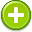correlate 中文解釋 wordnet sense Collocation Usage
Verb
/ˈkôrəˌlāt/,/ˈkär-/,Font size:correlated, past participle; correlated, past tense; correlating, present participle; correlates, 3rd person singular present;
1. Have a mutual relationship or connection, in which one thing affects or depends on another
• - the study found that success in the educational system correlates highly with class
2. Establish such a relationship or connection between
• - we should correlate general trends in public opinion with trends in the content of television news
Noun
1. Each of two or more related or complementary things
• - strategies to promote health should pay greater attention to financial hardship and other correlates of poverty

1. correlative: mutually related
2. to bear a reciprocal or mutual relation; "Do these facts correlate?"
3. either of two or more related or complementary variables
4. (correlation) a reciprocal relation between two or more things
5. correlation coefficient: a statistic representing how closely two variables co-vary; it can vary from -1 (perfect negative correlation) through 0 (no correlation) to +1 (perfect positive correlation); "what is the correlation between those two variables?"
6. (Correlation (projective geometry)) A correlation is a reciprocity (collineation from a projective space onto its dual space, taking points to hyperplanes and preserving incidence) with the identity as the associated automorphism.
7. (Correlated) Wallcoverings and fabrics designed to be used together. They are known as correlates or companions.
8. (correlated) Having a mutual or reciprocal relationship. Fitting together and varying together. See text, Chapter 13. See also, "coefficient of correlation," "mean," "regression" and "standard deviation."
9. (correlated) When observations are correlated, there are two or more observations (or derived quantities), which have at least one common source of error.
10. (correlated) two items that are related or connected are compared to one another.
11. (Correlation) Final state of the appraisal process in which appraiser reviews data and estimates property's value.
12. (Correlation) The relationship between two variables where change in one is associated with change in the other.
13. (correlation) A measure of association between two variables. It measures how strongly the variables are related, or change, with each other. If two variables tend to move up or down together, they are said to be positively correlated. ...
14. (Correlation) A measure of similarity between variables of functions.
15. (Correlation) A form of statistical modelling that attempts to summarise how one dataset will vary in response to another. A correlation coefficient of +1.0 means that where there are high values in one set there will be high values in the other, while a correlation coefficient of -1. ...
16. (Correlation) A statistical term that refers to a relationship between two seemingly independent things. In Forex for example, one could argue that the Euro and the Sterling have a higher correlation than, for example, the Euro and the Brazilian Real.
17. (Correlation) Statistical measure of the degree to which two variables move together in a linear fashion, either positively or negatively, that convertsCovariance to a standard scale ranging from -1 to +1.
18. (Correlation) A measure of the relationship between scores. Correlation scores vary between -1.0 and +1.0, with zero indicating no correlation. Scores that are measuring similar things, such as related items on scale or scores on scales measuring the same concept should be highly correlated. ...
19. (Correlation) The assocation of one message to another.
20. (CORRELATION) A basic level of learning where the student can associate what has been learned, understood, and applied with previous or subsequent learning.
21. (CORRELATION) HAVING THE SAME THINGS IN COMMON.
22. (CORRELATION) assuming linearity and homoscedasticity and both X and Y to be continuous (interval) data
23. (Correlation) A causal, complementary, parallel, or reciprocal relationship, especially a structural, functional, or qualitative correspondence between two comparable entities.
24. (Correlation) A cause-effect or if-then connection between two or more elements in a group of data.
25. (Correlation) A statistical term measuring the amount of similarity between the volatilities of any two indices, individual securities, or investment portfolios.